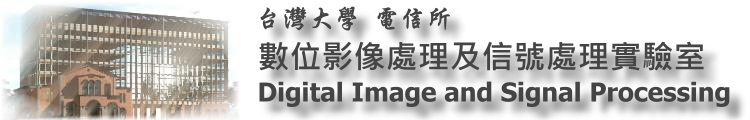[J.J Ding's PowerPoint File]

Publications

Presentation

Each person make a presentation of their research from time to time.

[Browse Contents] ← May have some new stuff that are not added above

Research areas

1. Fast Algorithm
• Integer Transform (entries are summations of 2k and reversible)
• Others (incl. Hankel transform, arbitrary point FFT, fractional transforms, etc.)
2. Fractional Transform (continuous and discrete form)
• Fractional Fourier Transform
• Filter Design
• Modulation and multiplexing
• Optical and radar system analysis
• Security, others
• Simplified fractional Fourier Transform
• Fractional Sine, Cosine, Hartley Transforms
• Fractional Hilbert Transform
• 1-D form, 2-D radial form
• Eigenfunction and eigenvector
• for FT
• for FT with offset
• for linear canonical transform
3. Time-Frequency analysis (continuous and discrete form)
• Wigner distribution function
• Wavelet
• Prolate Spheroidal Wave Function
• Gabor transform
4. Number theoretic transform
5. Image Processing
• Pattern Recognition
• N-D SVD
• Watermark, Encryption
• Corner and Edge Detection
6. Bioinformatics
• Sequence Alignment by DSP
• discrete correlation algorithm
• improved dynamic programming
• Sequence Assembly
7. Quaternion
8. Optical signal processing
• Amplitude-only (or phase only) Signal Processing
• Linear Canonical Transform
• Optical Communication
• Self-imaging phenomena (Hermite-Gaussian, Ince-Gaussian, fractal, etc.

Publication List

Introduction of Digital Signal Processing, Image Processing and some subareas of them